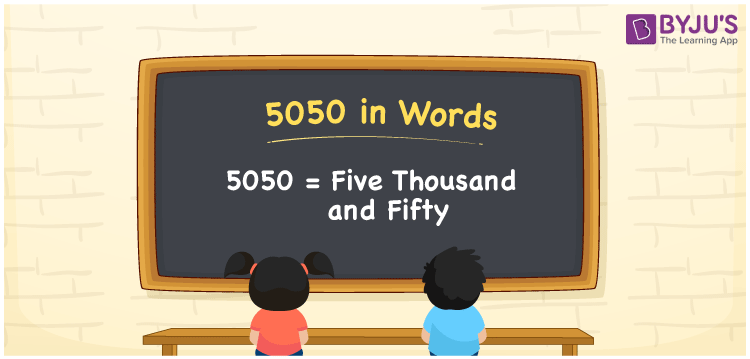# 5050 in words

5050 in words is written as Five Thousand and Fifty. 5050 represents the count or value. The article on Counting Numbers can give you an idea about count or counting. The number 5050 is used in expressions that relate to money, distance, length, year and others. Let us consider an example for 5050. “The distance between India and Korea is more than Five Thousand and Fifty Kilometers.”

 5050 in words Five Thousand and Fifty Five Thousand and Fifty in Numbers 5050

## 5050 in English Words## How to Write 5050 in Words?

We can convert 5050 to words using a place value chart. The number 5050 has 4 digits, so let’s make a chart that shows the place value up to 4 digits.

 Thousands Hundreds Tens Ones 5 0 5 0

Thus, we can write the expanded form as:

5 × Thousand + 0 × Hundred + 5 × Ten + 0 × One

= 5 × 1000 + 0 × 100 + 5 × 10 + 0 × 1

= 5050

= Five Thousand and Fifty.

5050 is the natural number that is succeeded by 5049 and preceded by 5051.

5050 in words – Five Thousand and Fifty.

Is 5050 an odd number? – No.

Is 5050 an even number? – Yes.

Is 5050 a perfect square number? – No.

Is 5050 a perfect cube number? – No.

Is 5050 a prime number? – No.

Is 5050 a composite number? – Yes.

## Solved Example

1. Write the number 5050 in expanded form

Solution: 5 × 1000 + 0 × 100 + 5 × 10 + 0 × 1.

We can write 5050 = 5000 + 50 + 0

= 5 × 1000 + 0 × 100 + 5 × 10 + 0 × 1.

## Frequently Asked Questions on 5050 in words

Q1

### How to write 5050 in words?

5050 in words is written as Five Thousand and Fifty.
Q2

### State whether True or False. 5050 is divisible by 3?

False. 5050 is not divisible by 3.
Q3

### Is 5050 divisible by 10?

Yes. 5050 is divisible by 10.